SVIBOR - Project code: 1-01-250

### MINISTRY OF SCIENCE AND TECHNOLOGY

Strossmayerov trg 4, HR - 10000 ZAGREB
tel.: +385 1 459 44 44, fax: +385 1 459 44 69
E-mail: ured@znanost.hr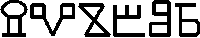## Probability Theory and Mathematical Statistics

### Main researcher: SARAPA, NIKOLA (42425)

Assistants
• BUTKOVIĆ, DAVOR (6293)
• JARDAS, CVETAN (80172)
• ELEZOVIĆ, NEVEN (57573)
• UGRIN-ŠPARAC, DIMITRIJE (51021)
• VONDRAČEK, ZORAN (113584)
• PENZAR, IVO (160994)
• UGRIN-ŠPARAC, GORDANA (109683)
• DEDIĆ, LJUBAN (98115)
• MATIĆ, MARKO (25774)
• ŠIKIĆ, HRVOJE (113595)
• ŠILAC, MIRTA (145672)
• HUZAK, MILJENKO (168846)
• BRNETIĆ, ILKO (136400)
• ŠTAMBUK, DEANA (900425)

Type of research: basic
Duration from: 01/01/91. to 12/31/95.

Papers on project (total): 71
Institution name: Prirodoslovno-matematički fakultet, Matematički odjel, Zagreb (37)
Department/Institute: Department of Mathematics, University of Zagreb
City: 10000 - Zagreb, Croatia
Communication

Summary: The investigations in this research project were concerned in the following areas: summability methods in probability theory and generalizations of the laws of large numbers; strongly regular matrices and their applications in probability theory; generalizations of classical ergodic type theorems and their applications in probability theory; ergodic type theorems for probability measures on groups and semigroups; extensions of finite measures; separability and uniform integrability of reversed martingales; inequalities of Kahane-Khintchine type in Orlicz space; uniform law of large numbers in the stationary case; the uniform ergodic theory; some generalizations of Haar measure; characterizations of Brownian motion and h-Brownian motion; the Martin kernel of positive harmonic functions; superprocesses and potential theory; stochastic investigations of pseudo-random number generators; some applications of probability and statistical methods in number theory; computer simulation of Gaussian random fields; Wiener random fields; generalizations of Wiener measure and Brownian motion; nonlinear perturbations of positive semigroups, some properties of generalized Schrodinger semigroups; gamma-potentials; investigations of Markov chains on infinite graphs; consistency of statistical models in the stationary case; exponential Orlicz norms; separability of families of reversed submartingales; uniform ergodic theorems for dynamical systems; randomly weighted series of contractions in Hilbert spaces; maximal inequalities for Bessel processes.

Keywords: summability methods, laws of large numbers, strongly regular matrices, martingales and reversed martingales, probability measures, weak convergence, ergodic type theorems, uniform ergodic theory, uniform integrability, Brownian motion, superprocesses and potential theory, extension of a measure, pseudo-radom number generators, Gaussian processes and fields, Wiener measure, pozitive semigroups, Schrodinger semigroup, gamma-potentials, Markov chains, statistical models, contraction, Bessel processes.

Research goals: The purpose of investigations in this scientific project is to obtain new and significant results in important fields in probability and mathematical statistics. We expect the concrete results in generalizations of laws of large numbers for pairs of sequences of random variables and random elements. These results are connected to the theory of summability of pairs of complex numbers and pairs of sequences in locally convex algebras. We shall stady the theory of strongly regular matrices and its applications in laws of large numbers, ergodic theory, Markov processes and probabilities on Banach spaces, topological groups and topological semigroups. By use of ergodic type theorems for bounded operators on Banach spaces we obtained limiting theorems in the sense of the weak topology for probability measures on compact semigroups. We expect new results in the uniform ergodic theory, in the theory of convergence of reversed martingales as in the asymptotic likelihood theory and consistency of statistical models. We also expect new results in the theory of Markov processes and corresponding potential theory as in the theory of superprocesses i.e. the processes with values in the set of measures. We try to characterize Brownian motion on some fractals by its exit distributions. We investigate some problems in connection with Hunt hypothesis. We shall study the problem of Radonification for cylindrical measures and F-cylindrical probabilities on tensor products of Banach spaces as the theory of random linear operators. We shall continue our investigations in statistical tests for valuation of generators of pseudo-random numbers. We also try to develop stochastic methods in foundation of new probabilistic number theory. We shall study Wiener random fields and some generalizations of Wiener measure. We shall investigate some problems about nonlinear perturbations of positive semigroups. We also investigate some properties of generalized Schrodinger semigroups and some problems about gamma-potentials. We expect new result in characterization of Markov chains on infinite graphs by limiting distributions. We also solve some problems about uniform ergodic theory for dynamical systems. We shall study the problem of consistency of statistical models in the stationary case and exponential Orlicz norms of stopped Brownian motion. We expect new results about separability of families of reversed submargingales and about uniform ergodic theorems for dynamical systems under VC entropy conditions. We shall study randomly weighted series of contractions in Hilbert spaces, some maximal inequalities of Kahane-Khintchine type in Orlicz spaces and some maximal inequalities for Bessel processes. We also solve problems about optimal stopping and maximal inequalities for geometric Brownian motion. The investigations in this project will last for approximately five years.

Other information about the project.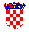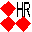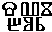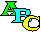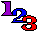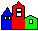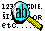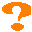Ministry of Science andTechnology Croatianlanguage Sviborhomepage Alphabeticlist Sorted onproject code Sorted oninstitutions Search Help

Last update: 10/16/95
Information: svibor@znanost.hr
